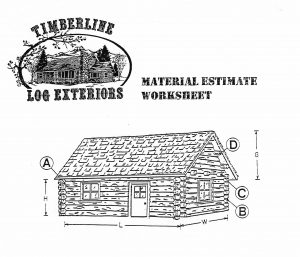# MeasuringLog Siding

• Determine the total area of exterior walls to be covered.                   _______sq.ft.
• Determine the area of the gable ends to be covered. (G x W x 1/2)  _______sq.ft.
• Subtract the area of windows, doors etc.                                           _______sq.ft.
• Add a waste factor of 8%                                                                   _______sq.ft.

Total Square Footage                                                                                  _______sq.ft.

Multiply the Total Square Footage _______ sq.ft. X 1.9 = _______ Linear ft. of log siding

This formula is for 2×8 log siding with a stack height of 6 1/2 ” to 6 5/8 “.

______________________________________________________________________

Log Corners

Determine the height of each outside corner in feet. Add all your corners together to determine the total footage of corners.

Total height of corners in feet______ X 3.75 =_____ log corners you will need.

example: 4 corners 8 ft. tall   32 ft X 3.75 = 120 log corners